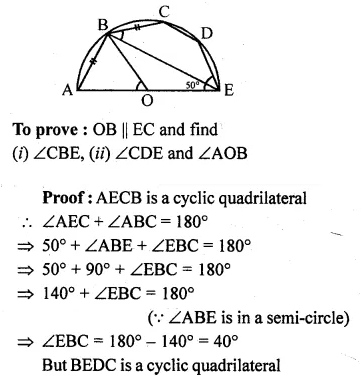Guru

# Question 12. In the given figure, O is the centre and AOE is the diameter of the semicircle ABCDE. If AB = BC and ∠AEC = 50°, find : (i) ∠CBE (ii) ∠CDE (iii) ∠AOB. Prove that OB is parallel to EC.

• 0

This is circle based question from Chapter name- circles
Topic – Angle properties of circles
Chapter number- 15

In the given figure, O is the centre and AOE is the diameter of the semicircle ABCDE.

AB = BC and ∠AEC = 50°,

Now we have to find : (i) ∠CBE (ii) ∠CDE (iii) ∠AOB.

Also we have to Prove that OB is parallel to EC.

ICSE Avichal publication
Understanding ICSE Mathematics
Question no 12

Share